# The Equation of Life

## Pupil Teacher Ratio

The equation links five quantities to the Pupil Teacher Ratio a school can afford in a balanced budget set for an academic year. The terms are defined below and the derivation of the equation is also given.

The equation is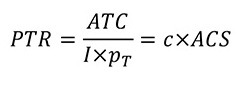Where

PTR is Pupil to teacher ratio

ATC is Average Teacher Cost

I is revenue available per pupil

PT is proportion of revenue available for expenditure on teacher cost

c is teacher contact ratio

ACS is average class size

There are other ways of calculating the number of teachers a school can afford to employ given estimates of funding, teaching salaries plus on cost and all other school expenditure but I have found the format above to make the calculation in terms of PTR is quite useful in a number of ways. Firstly, the quantities in the equation lend themselves easily to benchmarking activity. Secondly the link to the curriculum plan and hence the timetable through c and ACS is a simple one.

A third way of thinking of these terms is to estimate them for a given year and then view them as targets. Unexpected events such as long term illness or planned situations such as introducing increased management time change these targets and imply adjustment in other values in order to maintain a balance. The equation also provides a mechanism for looking at the finances from a non-financial point of view which also has the advantage of being in terms of only a few quantities.

Given a value for the PTR that the school can afford and a typical value for the teacher contact ratio the value of ACS can be calculated. Understanding the meaning of this number in the context of a school is a little like a doctor understanding the possible implications of a blood pressure value.

A value of ACS implies a budget of teacher periods for the curriculum plan which can be planned in the context of a staff deployment analysis.

Technical terms and basic relationships

Note that the relationships below apply to an academic year. If a school is working in financial years the values of ACS, I and pT should be estimated for the academic year that the teacher contact ratio, Pupil to Teacher Ratio and Average Class Size relate to

PTR

This is the pupil to teacher ratio found by dividing the pupil roll by the total number of teachers expressed in terms of Full Time Equivalent (FTE)

ATC

This the Average Teacher Cost and is the total salary plus on costs of all the teachers counted in PTR calculation divided by the FTE teaching staff total. It is probably useful to include supply and agency costs in addition to salary and on costs.

I

(The symbol is a capital letter I). This is the revenue funding in pounds per pupil. This is the sum of all funding, income and anything else which can be legitimately counted in the global pot of money to spend on revenue items divided by the number of pupils. I suggest that any carry forward is excluded so the in-year position can be assessed. If a school is working in financial years the value of I used should be estimated for the academic year in question.

pT

This is the proportion of revenue available for spending on teachers. It is the decimal fraction produced when the total revenue budget is divided into the sum of all expenditure lines not included in the ATC as defined above.

c

This is the teacher contact ratio. It is the average teaching load of the FTE teacher total used in the PTR and ATC calculations expressed as a fraction of the timetable cycle. It can be calculated by taking the total number of available teaching periods and dividing that by the product of the periods in the timetable cycle and the FTE teacher total used in earlier sections. It is typically a decimal in the 0.6 to 0.8 area. Values as high as 0.8 are now seem extremely rare. 0.8 was the norm around 30 years ago but school organisation now has quite different demands on it. I have only seen values for c as low as 0.6 in situations where schools are generously funded. More frequently, the values I have observed are in the mid-range between 0.7 and 0.8. For the last five years I have advocated 0.78 as an aspirational target on the basis that that represents approximately 10 per cent of all teacher time in planning and preparation, 10 per cent in management activity and allows 2 per cent error since 10+10+2 = 22 and 100-22 =78.

ACS

This is the average class size. This is a global statistic for an institution and does not actually represent any particular class. It is the number of pupils that would be allocated to a teacher if all the teachers who are teaching on any period of the week, on average shared all the pupils on roll evenly between them. There are two relationships for calculating it.

The first follows from the definition.

If tp represents the number of teacher contact periods on the whole timetable and w represents the number of periods there are in the timetable cycle then the average number of teachers in contact with students on any one period is given byIf the number of pupils on roll, N, is divided by this fraction we get the average class size so (N.B I have inserted multiplication signs to distinguish between separate symbols and symbols that have more than one letter.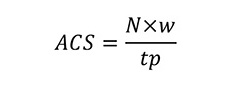The second is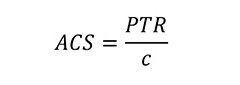This follows by substitution since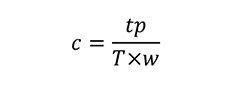where T is the full time equivalent number of teachers.

andThe second relationship for ACS is one of the two basic relationships needed to relate finance and curriculum (as above I have inserted multiplication signs from time to time to make the distinction between terms easier to see)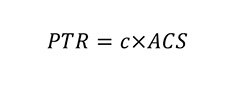The second basic relationship is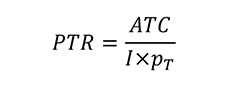This can be derived as follows:

Let the expenditure on teaching staff be £T

The average teacher cost ATC is therefore given by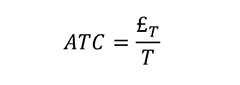So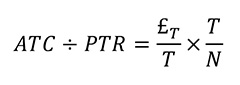So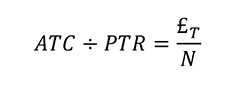But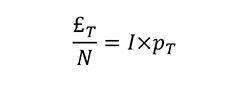Where I is the income per pupil and pT is the proportion of revenue expenditure on teaching staff

HenceSam Ellis
ASCL Funding Consultant

• Council
• Funding